### Addition of four digit numbers without carry

Question 1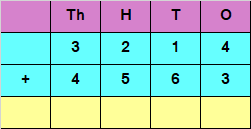Explanation

Adding the digits at ones place

4 + 3 = 7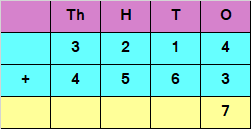Adding the digits at tens place

1 + 6 = 7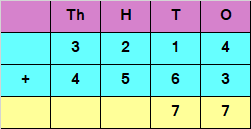Adding the digits at hundreds place

2 + 5 = 7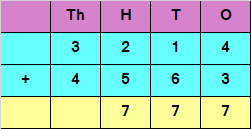Adding the digits at thousands place

3 + 4 = 7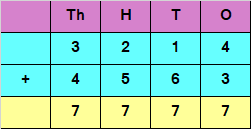Hence, the sum of the given numbers is 7777

Question 2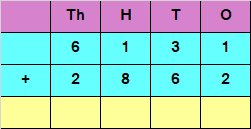Explanation

Adding the digits at ones place

1 + 2 = 3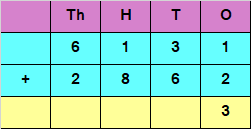Adding the digits at tens place

3 + 6 = 9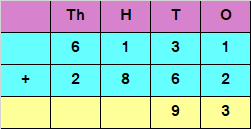Adding the digits at hundreds place

1  + 8 = 9Adding the digits at thousands place

6 + 2 = 8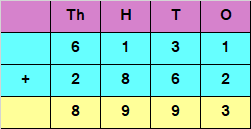Hence, the sum of the given numbers is 8993

### Addition of four digit numbers with carry

Question 1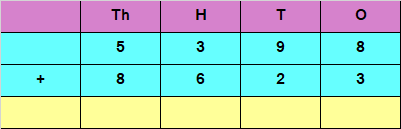Explanation

Adding the digits  at ones place ,

8 + 3 = 11

Since the number is more than 9, we  carry over 1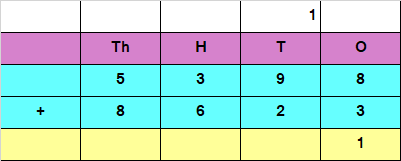Adding  the digits at tens place , and the carry over of 1

1 + 9 + 2  = 12

Since the number is more than 9, we  carry over 1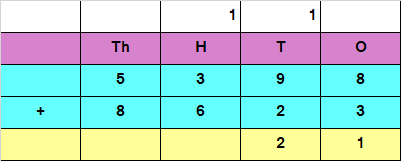Adding the digits at hundreds place, and the carry over of 1

1 + 3 + 6 = 10

Since the number is more than 9, we  carry over 1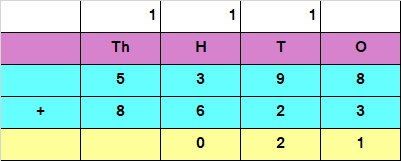Adding the digits at Thousands place, and the carry over of 1

Add 1 + 5 + 8  = 14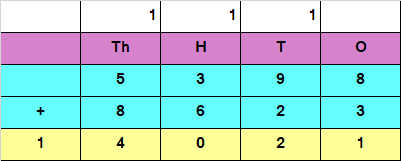Hence, the sum of the given numbers  is 14021

Question 2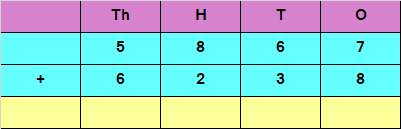Explanation

Adding the digits at ones place ,

7 + 8 = 15

Since the number is more than 9, we  carry over 1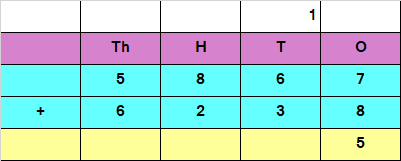Adding  the digits at tens place , and the carry over of 1

1 + 6 + 3 = 10

Since the number is more than 9, we carry over 1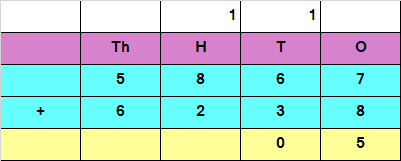Adding the digits at hundreds place, and the carry over of 1

1 + 8 + 2 = 11

Since the number is more than 9, we carry over 1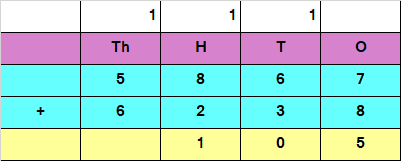Adding  the digits at Thousands place, and the carry over of 1

Add 1 + 5 + 6 = 12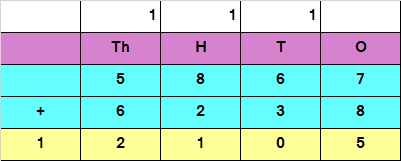Hence, the sum of the given numbers  is 12105